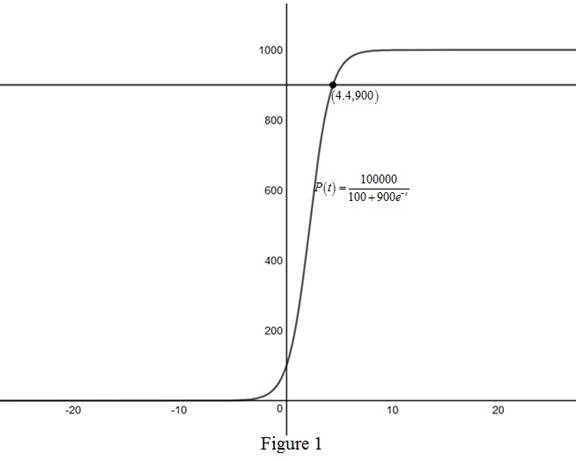# To estimate: The time taken in which the population reaches 900 with the help of graph of the given equation.### Single Variable Calculus: Concepts...

4th Edition
James Stewart
Publisher: Cengage Learning
ISBN: 9781337687805### Single Variable Calculus: Concepts...

4th Edition
James Stewart
Publisher: Cengage Learning
ISBN: 9781337687805

#### Solutions

Chapter 1, Problem 28RE

(a)

To determine

## To estimate: The time taken in which the population reaches 900 with the help of graph of the given equation.

Expert Solution

Solution:

The time taken in which the population to reach 900 is approximately 4.4 years.

### Explanation of Solution

Given:

The initial population in an limited environment is 100 with the carrying capacity 1000.

Also the population at any instant of time t is, P(t)=100,000100+900et.

Graph:

Use online graph calculator and draw the graph of the function P(t)=100,000100+900et when P(t)=900.From Figure1,obtain that point of intersection of the graph of P(t)=100,000100+900et and the line y=900 is (4.4,900).

Therefore, time taken by the population to reach 900 is approximately 4.4 years.

(b)

To determine

### To find: The inverse of the given function and to explain its meaning.

Expert Solution

Solution:

The inverse of the function is t=ln[9P1000P] and the meaning of this inverse function is the time taken in which the population to reach P.

### Explanation of Solution

Calculation:

Let P(t)=P.

P=100,000100+900et100+900et=100,000P900et=100,000P100et=100.000900P100900

Again solve the above equation,

et=10009P19=10009PP9P1et=1000P9P

Take reciprocal on both sides,

et=9P1000P

Take natural logarithm on both sides,

lnet=ln(9P1000P)

Since lnet=t,

Therefore, t=ln[9P1000P].

Where t is the inverse of the function and t denotes the time taken by the population to reach P.

(c)

To determine

### To find: The time required by the population to reach 900 by using the inverse function.

Expert Solution

Solution:

The time required for the population to reach 900 by using the inverse function is approximately 4.39 years.

### Explanation of Solution

Calculation:

From part(b), the inverse of the function is t=ln[9P1000P].

Given that P=900.

Therefore, the inverse of the function is,

t=ln[9(900)1000900]=ln=ln(81)t4.39

Thus, the time required for the population to reach 900 by using the inverse function is approximately 4.39 years.

Compare this answer with the answer in part (b) and observe that the answers are approximately same.

### Have a homework question?

Subscribe to bartleby learn! Ask subject matter experts 30 homework questions each month. Plus, you’ll have access to millions of step-by-step textbook answers!